# REFERENCE MANUAL

SOLVESPACE is a (GPLv3) parametric 3D CAD tool developed by Johnathan Westhues and other authors from 2008-2023. PrintNow extended SolveSpace in 2023 to help our Enterprise customers take control of the first open-source CAD tool designed specifically for eCommerce print packaging.

## User Interface

The user interface consists of two windows: a larger window containing mostly graphics and a smaller one containing mostly text. The graphics window is used to draw the geometry and to view the 2d structure. The text window provides information about the structure and may also be used to modify settings and numerical parameters.

### Graphics Window and Model View

To pan the view, right-drag with the mouse. It is also possible to pan by Shift+center-dragging.

To zoom in or out, rotate the scroll wheel. Zooming using the View menu or the associated keyboard shortcuts (+ and -) is also possible. Some features, including the planes, are always drawn the same size on-screen and are therefore not affected by zooming.

Most commands are available in three ways: from a menu, keyboard shortcut, or toolbar. The toolbar is displayed at the top left of the graphics window. To learn what an icon means, hover the mouse over it. To show or hide the toolbar, choose View → Show Toolbar.

To zoom to the extent of the part, choose View → Zoom To Fit. This adjusts the zoom level to fit precisely on the screen, then pans to center the structure.

If a workplane is active, choose View → Align View to Workplane (or press W) to align the view to the workplane. After doing this, the plane of the screen is coincident with the workplane, and the center of the workplane is at the center of the screen. The zoom level is not affected.

To pan the view so that a given point is at the center of the screen, select that point and then choose View → Center View at Point.

### Dimension Entry and Units

Dimensions are mainly displayed in either millimeters or inches. Millimeter dimensions are always displayed with two digits after the decimal point (45.23), and inch dimensions are always shown with three (1.781).

Choose View → Dimension Units → Dimensions in Inches/Millimeters to change the current display units. This does not change the model; if the user changes from inches to millimeters, a dimension entered as 1.0 is now displayed as 25.40.

All dimensions are entered in the current display units. In most places where a dimension is expected, it's possible to enter an arithmetic expression ("4*20 + 7") instead of a single number.

You can use sqrt, square, sin, cos, asin, acos, pi, +, -, *, /, (, ) in the expressions. For example "7*pi/(3+cos(45))". The trigonometric functions take degrees.

In addition, you can use in, mm, and m in the expressions to convey absolute unit values (inches, millimeters, and meters, respectively). For example, "width*2+in(4)".

### Property Browser

The text window appears as a floating palette window. It may be shown or hidden by pressing Tab or View → Show Text Window.

The text window works like a web browser. Any underlined text is a link. To activate a link, click it with the mouse. The links may be used to navigate other pages in the text window. For example, the "home" screen is a list of groups in the sketch:To navigate a group's page, click that group's name (e.g., "g002-sketch-in-plane"). The links may also trigger actions in the sketch. For example, in the above screenshot, all groups are shown. To hide a group, click the box in the "shown" column.

As a convenience, the text window will automatically navigate to a page that is likely to be relevant. For example, when a new group is created, the text window displays that new group's page. Navigating to a different page is always possible by clicking the "home" link at the top left corner (or pressing Esc) and following the links.

When sketch entities are selected (e.g., the user has clicked on them with the mouse), information about those entities is displayed in the text window. If a single entity is selected, then information about that entity is displayed. For example, the window display's a circle's center and radius.

If multiple entities are selected, the text window can sometimes display information about all of them. These cases include:

• two points: the distance between the points
• a point and a plane face: the distance from the point to the plane
• two points, and a vector: the distance between the points, projected along the vector
• two plane faces: the angle between the plane faces

## Sketch Entities

###Datum Point (P)

A single point defines this entity.

If a workplane is active when the datum point is created, then that point will always lie in the workplane. This is the same behavior for all points, e.g., the endpoints of a line segment.

Points are typically used as construction geometry. The user might place datum points to simplify the dimensioning of line segments or other entities.

###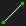Line Segment (S)

This entity is specified by its two or more connected points. If a workplane is active, the two endpoints will always lie in that workplane.

To create a Line Segment:

1. Choose Sketch ‣ Line Segment
2. Left-click to mark the starting point of the line segment.
3. Release the mouse button. The endpoint is now being dragged.
4. To create another line segment that shares an endpoint with the line segment that was just created, left-click again.
5. Press Esc or right- or middle-click with the mouse to stop drawing line segments.

SolveSpace will also stop drawing new line segments if an automatic constraint is inserted.

To close a line segment into a polygon:

Hovering over the starting point before left-clicking the last time. The endpoint of the polyline will be constrained to lie on the starting point, and since a constraint was inserted, SolveSpace will stop drawing.

###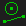Construction Line Segment (Shift+S)

In normal operation, the user draws lines and curves in a sketch. Those curves describe the geometry to be manufactured; ultimately, the endmill, the laser, or some other tool will cut along those curves.

In some cases, drawing a line that should not appear in the final part is helpful. For example, the user may wish to draw a center line for a symmetric structure; but that center line is only a guide and should not get exported with the CAM data. These lines are called construction lines.

To mark an entity as construction-only, choose Sketch → Toggle Construction. A construction entity will behave just like any other entity, except that it is drawn in green and does not contribute to the geometry for export by default (or to the section that will be extruded, lathed, or swept). You may also toggle construction geometry while sketching a new entity using the 'g' keyboard shortcut.

###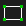Rectangle (R)

This entity comprises two vertical line segments and two horizontal line segments arranged to form a closed curve. Initially, the rectangle is specified with the mouse by two diagonally opposite corners. The rectangle's line segments (and points) may be constrained like ordinary line segments.

It would be possible to draw the exact figure by hand by drawing four line segments and inserting the appropriate constraints. The rectangle command is a faster way to draw the very same thing.

A workplane must be active when the rectangle is drawn since the workplane defines the meaning of "horizontal" and "vertical."

###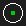Circle (C)

This entity is specified by its center point, diameter, and normal.

To create the circle, choose Sketch → Circle and left-click the center. Then release the mouse button; the circle's diameter is now being dragged. Left-click again to place the diameter.

If a workplane is active, the center point must lie in that workplane, and the circle's normal is parallel to the workplane's normal (which means that the circle lies in the plane of the workplane).

###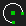Arc of a Circle (A)

This entity is specified by its center point, the two endpoints, and its normal.

To create the arc:

1. Choose Sketch ‣ Arc of a Circle.
2. Left-click one of its endpoints.
3. Then release the mouse button; the other endpoint is now being dragged. The center is also being dragged in such a way as to form an exact semi-circle.
4. Left-click again to place the other endpoint.
5. Drag the center to the desired position. The arc is drawn counter-clockwise from the first point to the second.

The arc must be drawn in a workplane; it cannot be drawn in free space.

###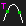Tangent Arc at Point (Shift+A)

To round off a sharp corner (for example, between two lines), we often wish to create an arc tangent to both lines at the corner. This will create a smooth appearance where the line and arc join. It would be possible to draw these arcs by hand, using Sketch → Arc of a Circle and Constrain → Tangent, but it's easier to create them automatically.

1. Select a point where two line segments or circles join.
2. Choose Sketch ‣ Tangent Arc at Point. The arc will be created and automatically constrained to the two adjoining curves.

The initial line segments will become construction lines, and two new lines will be created that join up to the arc. The arc's diameter may then be constrained in the usual way, with Distance / Diameter or Equal Length / Radius constraints.

By default, the radius of the tangent arc is chosen automatically. To change that, choose Sketch → Tangent Arc at Point with nothing selected. A screen will appear in the text window, where the radius may be specified. It is also possible to specify whether the original lines and curves should be kept but changed to construction lines (which may be helpful if you want to place constraints on them) or delete them.

###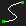Bezier Cubic Spline (B)

At least two on-curve points specify this entity and an off-curve control point at each end (so two off-curve points total). If only two on-curve points are present, this is a Bezier cubic section, and the four points are exactly the Bezier control points.

If more on-curve points are present, it is a second derivative continuous (C2) interpolating spline composed of multiple Bezier cubic segments. This is a useful type of curve because it has a smooth appearance everywhere, even where the sections join.

To create the Bezier cubic spline:

1. Choose Sketch ‣ Bezier Cubic Spline.
2. Left-click one endpoint of the cubic segment.
3. Release the mouse button. The other endpoint of the cubic segment is now being dragged.
4. To add more on-curve points, left-click with the mouse.
5. To finish the curve, right-click, or press Esc.

The two control points are initially placed on the straight line between the endpoints; this means that the cubic initially appears as a straight line. Drag the control points to produce the desired curve.

To create a closed curve (technically, a "periodic spline"), start by creating the curve as usual, left-clicking to create additional on-curve points. Then hover the mouse over the first point in the curve, and left-click. The curve will be converted to a periodic spline, C2 continuous everywhere, including at that first point.

###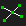Splitting and Trimming Entities (I)

In some cases, drawing is desirable by creating overlapping figures and removing the extra lines. For example, a circle and a rectangle are drawn; the two short lines and the short arc are then deleted to form a single closed shape.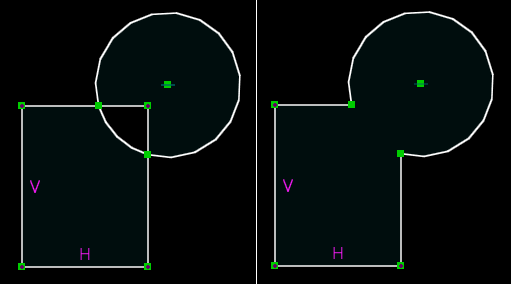To trim the extra lines, splitting the entities where they intersect is necessary. SolveSpace can split lines, circles, arcs, and Bezier curves against each other. To do so, select the two entities to be split, then choose Sketch → Split Curves at Intersection. This deletes each original entity and replaces it with two new entities that share an endpoint at the intersection. The excess lines may then be deleted as usual.

Because the original entities are deleted, any constraints on the original entities are deleted as well. This means that the sketch may no longer be constrained as desired after splitting. If an entity is marked as construction before splitting, it will not be deleted, so that the constraints will persist.

## Constraints

### General

To create a constraint, first, select the geometry to be constrained. For example, when constraining the distance between two points, first select those two points. Then choose the appropriate constraint from the Constrain menu.

The same menu item may generate different constraints depending on what is selected. For example, the Distance / Diameter menu item will generate a diameter constraint if a circle is selected but a length constraint if a line segment is selected. If the selected items do not correspond to an available constraint, then SolveSpace will display an error message and a list of available constraints.

If a workplane is active, then the constraint applies to the geometry projection into that workplane. If no workplane is active, then the constraint applies to the actual geometry in free space.

Constraints are drawn in purple on the sketch. If a constraint has a label associated with it (e.g., a distance, variable, or an angle), then that label may be repositioned by dragging it with the mouse. To modify the dimension, double-click the label; a text box will appear on the screen, where the new value can be entered. Press enter to commit the change or Esc to cancel.

### Failure to Solve

In some cases, the solver will fail. This is usually because the specified constraints are inconsistent or redundant. For example, a triangle with internal angles of 30, 50, and 90 degrees is inconsistent--the angles don't sum to 180, so the triangle could never be assembled. This is an error.

A triangle with internal angles constrained to 30, 50, and 100 degrees is also an error. This is not inconsistent because the angles sum to 180 degrees, but it's redundant because only two of those angles need to be specified.

If the sketch is inconsistent or redundant, then the background of the graphics window is drawn in red (instead of the usual black), and an error is displayed in the text window:As a convenience, SolveSpace calculates a list of constraints that could be removed to make the sketch consistent again. To see those constraints, hover the mouse over the links in the text window; the constraint will appear highlighted in the graphics window. The user can make the sketch consistent again by deleting one or more of the constraints in that list.

A different type of error occurs when the solver fails to converge. This may be a defect in the solver, or it may occur because impossible geometry was specified (for example, a triangle with side lengths 3, 4, and 10; 3 + 4 = 7 < 10). A similar error message is displayed without a list of constraints to remove to fix things. The problem can be resolved by removing or editing the constraints or choosing Edit → Undo.

### Reference Dimensions

By default, the dimension drives the geometry. If a line segment is constrained to have a length of 20.00 mm, then the line segment is modified until that length is accurate.

A reference dimension is the reverse: the geometry drives the dimension. If a line segment has a reference dimension on its length, it's still possible to freely change that length, and the dimension displays whatever that length is. A reference dimension does not constrain the geometry.

To convert a dimension into a reference dimension, choose Constrain → Toggle Reference Dimension. A reference dimension with "REF" is drawn to the displayed length or angle. Double-clicking a reference dimension does nothing; the dimension is specified by the geometry, not the user, so it is not meaningful to type in a new value for the reference dimension.

### Specific Constraints

To get help on a specific constraint, choose its menu item without selecting any entities. An error message will be displayed, listing all of the possibilities.

Generally, the order in which the entities are selected doesn't matter. For example, if the user is constraining point-line distance, they might select the point, the line, or the line and then the point, and the result would be identical. Some exceptions exist and are noted below.

###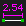Distance / Diameter (D)

This constraint sets the diameter of an arc or a circle, the length of a line segment, or the distance between a point and some other entity.

The distance is signed when constraining the distance between a point and a line in a workplane.

### Reference Dimensions

By default, the dimension drives the geometry. If a line segment is constrained to have a length of 20.00 mm, then the line segment is modified until that length is accurate.

A reference dimension is the reverse: the geometry drives the dimension. If a line segment has a reference dimension on its length, it’s still possible to freely change that length, and the dimension displays whatever that length is. A reference dimension does not constrain the geometry.

To convert a dimension into a reference dimension:

• Choose Constrain ‣ Toggle Reference Dimension.

A reference dimension is drawn with ‘REF’ to the displayed length or angle. Double-clicking a reference dimension does nothing; the dimension is specified by the geometry, not the user, so it is not meaningful to type in a new value for the reference dimension.

###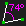Angle (N)

This constraint sets the angle between two vectors. A vector is anything with a direction; in SolveSpace, line segments and normals are vectors. (The constraint could apply to two line segments, a line segment and a normal, or two normals.) The angle constraint is available in both projected and 3d versions.

The angle must always lie between 0 and 180 degrees. Larger or smaller angles may be entered but will be taken modulo 180 degrees. The sign of the angle is ignored.

When two lines intersect, four angles are formed. These angles form two equal pairs. For example, the pictured lines intersect at 30 degrees and 150 degrees. These two angles (30 and 150) are known as supplementary angles and always sum to 180 degrees.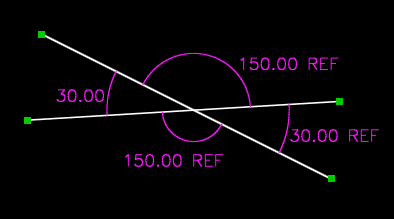(Notice that three of the angle constraints are reference dimensions in the sketch. Given any one of the angles, we could calculate the other three, so a sketch that specified more than one of those angles would be overconstrained and fail to solve.)

When a new angle constraint is created, SolveSpace chooses arbitrarily which supplementary angle to constrain. An arc is drawn on the sketch to indicate which angle was chosen. As the constraint label is dragged, the arc will follow.

If the wrong supplementary angle is constrained, select the constraint and choose Constrain → Other Supplementary Angle. A constraint of 30 degrees on one supplementary angle is equivalent to 150 degrees on the other.

###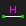Horizontal (H) /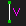Vertical (V)

This constraint forces a line segment to be horizontal or vertical. It may also be applied to two points, which applies to the line segment connecting those points.

A workplace must be active because the meaning of "horizontal" or "vertical" is defined by the workplane.

It's good to use horizontal and vertical constraints whenever possible. These constraints are simple to solve and will not lead to convergence problems. Whenever possible, define the workplanes so that lines are horizontal and vertical within those workplanes.

###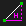Horizontal Projection (Shift + H) /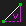Vertical Projection (Shift +V)

Horizontal and vertical projection allows you to apply a constraint between any two points.

###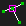On Point / Curve / Plane (O)

This constraint forces two points to be coincident, a point to lie on a curve or a point to lie on a plane.

The point-coincident constraint is available in projected versions. The projected version restricts two degrees of freedom. When a point is constrained to lie on a circle (or an arc of a circle), the actual constraint forces the point to lie on the cylindrical surface through that circle.

###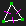Equal Length / Radius / Angle (Q)

This constraint forces two lengths, angles, or radiuses to be equal.

The equal-angle constraint requires four vectors as input: the two equal angles are the angle between each pair of inputs. For example, select line segments A, B, C, and D. The constraint forces the angle between lines A and B to be equal to the angle between lines C and D. If the wrong supplementary angle is chosen, then choose Constrain → Other Supplementary Angle, as for the angle constraint.

If a line and an arc of a circle are selected, then the length of the line is forced equal to the length (not the radius) of the arc.

### Length Ratio (Z)

This constraint sets the ratio between the lengths of two line segments. For example, if lines A and B have a length ratio of 2:1, then the constraint is satisfied if A is 50 mm long and B is 25 mm long.

The order in which the lines are selected matters; if line A is selected before line B, then the ratio is the length of A's length: B.

### Length Difference (J)

This constraint sets the difference between the lengths of two line segments. For example, if lines A and B have a length difference of 5 mm, then the constraint is satisfied if A is 50 mm long and B is 55 mm long or 45mm long.

Note that a negative difference can be entered when editing the constraint to change, which is the shorter segment. At the same time, the value is always displayed as a positive number (the absolute difference), and entering positive numbers does not change the "direction."

### At Midpoint (M)

This constraint forces a point to lie on the midpoint of a line.

The at-midpoint constraint can also force the midpoint of a line to lie on a plane; this is equivalent to creating a datum point, constraining it at the midpoint of the line, and then constraining that midpoint to lie on the plane.

###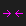Symmetric (Y)

This constraint forces two points to be symmetric about some plane. Conceptually, if we placed a mirror at the symmetry plane and looked at the reflection of point A, it would appear to lie on top of point B.

The symmetry plane may be specified explicitly by selecting a workplane. Or, the symmetry plane may be specified as a line in a workplane; the symmetry plane is then through that line and normal to the workplane. Or, the symmetry plane may be omitted; in that case, it is inferred to be parallel to either the active workplane's vertical axis or its horizontal axis. The horizontal or vertical axis is chosen, depending on which is closer to the configuration in which the points were initially drawn.

###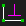Perpendicular ([)

This constraint is exactly equivalent to an angle constraint for ninety degrees.

###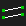Parallel / Tangent (L)

This constraint forces two vectors to be parallel.

A zero-degree angle constraint is equivalent to a parallel constraint in 2d (i.e. when a workplane is active).

This constraint can also force a line to be tangent to a curve or force two curves (for example, a circle and a cubic) to be tangent to each other. The two curves must already share an endpoint to do this, usually achieved with a point-coincident constraint. The constraint will force them to also be tangent at that point.

### Lock Point Where Dragged (])

Constrain a point such that the solver will not alter its location. This does not prevent direct manipulation by dragging the entity that owns the point or itself. It simply instructs the solver to consider the point fully constrained. This can be easily demonstrated by drawing an equilateral triangle with sides constrained equally and one point locked. Dragging the side opposite this locked point will not alter its position but will resize the triangle.

### Show Underconstrained Points (Ctrl+Shift+S)

This feature indicates which points in the sketch are not completely constrained (i.e., which points would move if the user dragged them with the mouse). The free points are drawn with a large cyan square over them. In a sketch with zero degrees of freedom, nothing would be drawn. In a sketch with one degree of freedom, one or more points would be marked; it's possible that more than one point would be marked since that one degree of freedom might be draggable via multiple points.

### Comment (;)

A comment is a single line of text that appears on the drawing. To create a comment, select Constrain → Comment, then left-click the center of the new comment. To move the comment, drag it with the mouse. To change the text, double-click it.

The comment does not affect the geometry; it is only a human-readable note. By default, the comment is shown in the same color as the constraints; but a comment may be assigned to a custom line style to specify the line width and color, text height, text origin (left, right, top, bottom, center), and text rotation.

If a comment is created within a workplane, then the text will be drawn within the plane of that workplane. Otherwise, the text will always be drawn facing forward, in the plane of the screen.

By default, comments are hidden when their group is inactive, as for all other constraints. If a comment is assigned to a custom style, it will be shown even when its group is inactive, as long as the style (and the group and constraints overall) is shown.

## Line Styles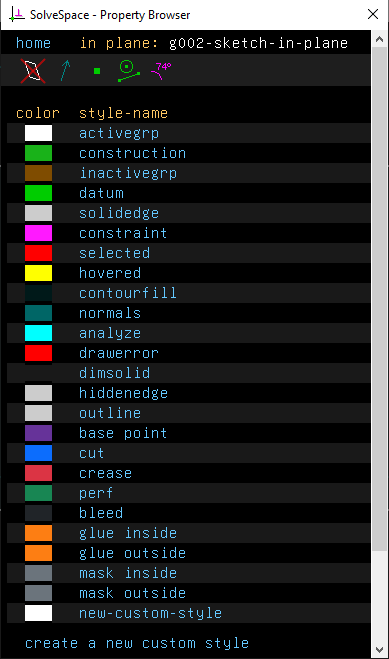It is never possible to directly change a line or curve's color, line width, or other cosmetic properties. Instead, the cosmetic properties of an entity may be specified by assigning that entity to a line style. The style specifies color, line width, text height, text origin, text rotation, and certain other properties that determine how (and whether) an object appears on-screen and in an exported file.

SolveSpace's basic color scheme is defined by the default styles. To view them, choose "line styles" from the home screen in the text window. This is where, for example, lines are specified to be white by default, constraints to be magenta, and points to be green. The default styles may be modified. These modifications will be saved in the user config (the registry on Windows, a .json file on other platforms) and applied to all files opened on that computer.

It is also possible to create custom styles for cosmetic or other purposes. (For example, laser cutters often use the color of a line to specify the power and feed with which to cut it.) Choose "new custom style" on the style screen to create a custom style. The new style will appear at the bottom of the list, with the default name new-custom-style.

To modify a style, click its link in the list of styles. The Color is red, green, and blue, where each component is between 0 and 1. The line width may be specified in pixels, inches, or millimeters. If the line width is specified in pixels, then the line width in an exported file will depend on the on-screen zoom level. If the user zooms out (so that the model appears smaller on screen), then the geometry shrinks, but the lines, measured in pixels, stay the same size; this means that lines will appear thicker than the model in the exported file.

Entities (like lines, circles, or arcs) may be assigned to a line style. One way to do so is to enter the desired line style's screen in the text window and select the desired entities. Then click the "assign to style" link in the text window. Another way to do so is to right-click the entity and assign it to the style using the context menu.

Comments (Constrain → Comment) may also be assigned to styles. The style may be used to specify the text height, the rotation of the text in degrees, and from what point (top, bottom, left, right, center) the text is aligned. The snap grid (View → Show Snap Grid, Edit → Snap to Grid) is often useful when creating cosmetic text.

User-created styles are saved in the .slvs file, along with the geometry. If a part with user-created styles is linked in, then the styles are not imported; but the style identifiers for the linked-in entities are maintained. This means that the user can specify the line styles in the file doing the linking (i.e., the "assembly").

If a style is hidden, all objects within that style will be hidden, even if their group is shown. If a style is not marked exportable, then objects within that style will appear on-screen but not in an exported file. This behavior is similar to that of construction lines.

## Variables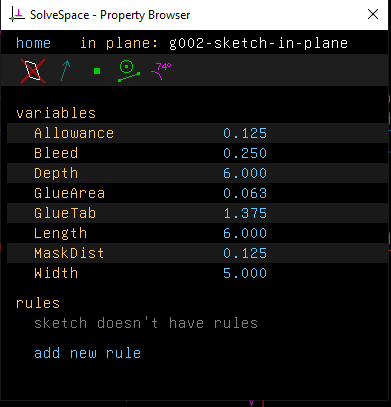Variables are used when engineering print parametric standards in SolveSpace 2D. Any dimension can change to a variable by double-clicking the dimension label and entering the variable name. Doing so will prompt the user to enter a default value that can change anytime within the SolveSpace 2D application or be overridden within the PrintNow platform set-up.

### Rules

Rules can be attached along with variables to prevent a standard from resolving within the SolveSpace 2D application. Any saved rule will be automatically added to the PrintNow platform on upload, preventing the user from ordering unless it’s allowed. When clicking “add new rule” on the Variables screen, any Expression can be added, as well as the Error message you would want to display when the rule should display. Examples of expression that includes variables with some equations are:

2*Width+3*Depth<=in(47)

Length+2*Width<=in(47)

Length>=Width

Width>=Depth

## Expression System

Expressions in SolveSpace 2D have syntax that matches most C-style languages such as C, C++, C#, Java and JavaScript. In addition to the syntax being similar the operator precedence is also the same meaning that people who have done any coding in those languages should be comfortable creating/editing expressions in SolveSpace.

### Math Operators

• +   Adds two numeric values. Ex: Width + 10
• -   Subtracts two numeric values. Ex: Width - 10
• *   Multiplies two numeric values. Ex: Width * 2
• /   Divides two numeric values. Ex: Width / 2

### Sub-expression Operators

• (   Identifies the beginning of a sub-expression. Ex: Width + (Allowance * 2)
• )   Identified the end of a sub-expression. Ex: Width + (Allowance * 2)

### Comparison Operators

• ==   Returns true if two values are equal. Ex: Width == 12
• !=   Returns true if two values are not equal. Ex: Width != 12
• <    Returns true if a value is less than another. Ex: Width < 12
• <=   Returns true if a value is less than or equal to another. Ex: Width <= 12
• >    Returns true if a value is greater than another. Ex: Width > 12
• >=   Returns true if a value is greater than or equal to another. Width >= 12
• ||   Return true if at least one condition is true. Ex: Width < 8 || Width > 12
• &&   Return true if conditions on both sides are true. Ex: Width > 8 && Width < 12

### Conditional Operators

• ? and :   Creates a conditional expression that can return one of two values based on whether a condition is true or false.. Must be used together. Ex: Width < 6 ? Padding : Padding * 2

### Math functions/constants

• abs Returns the absolute value of a numeric value. Ex: abs(Width - 10)
• sqrt Returns the square root of a numeric value. Ex: sqrt(Width * Width + Height * Height)
• square Returns the square of a numeric value. Ex: square(Width) + square(Height)
• sin Returns the sine of a numeric value (angle in degrees). Ex: sin(Angle + 45)
• cos Returns the cosine of a numeric value (angle in degrees). Ex: cos(Angle + 45)
• floor Return the nearest whole number less than or equal to a numeric value. Ex: floor(Width / 2)
• ceil Returns the nearest whole number greater than or equal to a numeric value. Ex: ceil(Width / 2)
• pi Returns the value of PI. Ex: pi * square(Radius)
• mm Returns a numeric value as if it were millimeter units without regard to what the working units are in SolveSpace. Ex: mm(254)
• in Returns a numeric value as if it were inch units without regard to what the working units are in SolveSpace. Ex: in(10)
• m Returns a numeric value as if it were meter units without regard to what the working units are in SolveSpace. Ex: m(0.254)

• min Returns the smallest of the numeric values passed in.

Syntax definition: min(num1, num2 [... , numN])   Ex: min(Width / 2, Length)

• max Returns the largest of the numeric values passed in.
• Syntax definition: max(num1, num2 [... , numN])   Ex: max(Width / 2, Length)
• clamp Returns a numeric value clamped to a specific min and max range.

Syntax definition: clamp(value, minLimit, maxLimit)   Ex: clamp(Width, 50.8, 101.6)

• step Returns a numeric value based on a numeric value compared to 1 or more breakpoints.

Syntax definition: step(value, default, limit1, value1 [..., limitN, valueN])   Ex: step(Allowance, 0, 1, .79375, 3, 1.5875, 4, 3.175) as

Based on the example above the step function will return:

• 0 until Allowance is greater than or equal to 1
• .79375 until Allowance is greater than or equal to 3
• 1.5875 until Allowance is greater than or equal to 4
• 3.175 when Allowance is greater than or equal to 4

### Operator Precedence

Below is a list of the operators in precedence order.

• Sub-expression operators
• Math functions
• Multiplication and division operators
• Comparison operators
• Conditional operators
• Constants, numbers and parametric variables

## Sample Files

### 03-Economy-min.slvs

This file is intended to focus on the min function, specifically showing how min can be used in place of a conditional in some cases.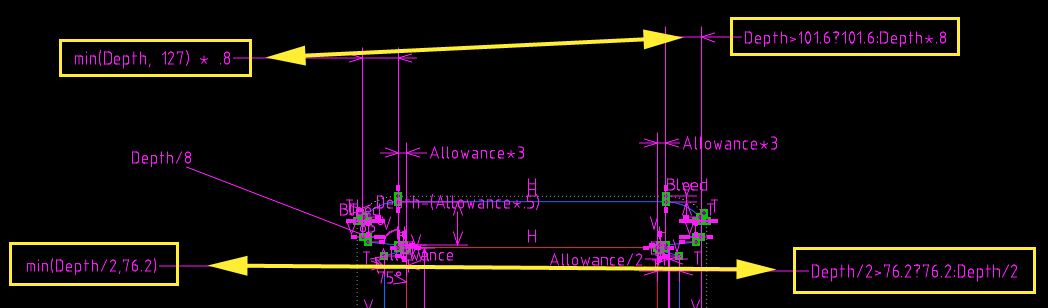### 04-Self-Locking-OPF-step.slvs

This file is intended to show how a complex nested conditional expression can be simplified by using the step function.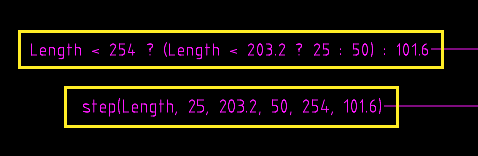### DH-clamp.slvs

This file is intended to illustrate how the clamp function might be used. Changing Width and Height variables allows you to see how the clamp function can limit a value to a certain range.

### F0200-min.slvs

This file is intended to show how using the min function could offset the need to implement certain restrictions in your file (such as requiring that Width <= Length for example).

### Min-max-clamp-step-playground.slvs

This file is intended to help understand the output of each function in a simple way. Change the Width variable to see how each of the lines are affected by the various functions.

0 out of 0 found this helpful